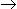# Chemical Engineering - Chemical Reaction Engineering

### Exercise :: Chemical Reaction Engineering - Section 10

6.

Pick out the correct statement.

 A. A chemical reaction accompanied by absorption of heat is called an exothermic reaction. B. A chemical reaction accompanied by evolution of heat is called an endother-mic reaction. C. The rate constant for a first order reaction does not change on changing the concentration units. D. Chemical equilibrium state is dynamic in nature.

Explanation:

No answer description available for this question. Let us discuss.

7.

The concentration of A in a first order reaction, AB, decreases

 A. linearly with time. B. exponentially with time. C. very abruptly towards the end of the reaction. D. logarithmically with time.

Explanation:

No answer description available for this question. Let us discuss.

8.

The equilibrium constant of chemical reaction __________ in the presence of catalyst.

 A. increases B. decreases C. remains unaffected D. can either increase or decrease (depends on the type of catalyst)

Explanation:

No answer description available for this question. Let us discuss.

9.

The order of a chemical reaction is

 A. an experimentally determined quantity. B. always equal to the total stoichiometric number of reactants. C. never fractional. D. none of these.

Explanation:

No answer description available for this question. Let us discuss.

10.

A chemical reaction occurs, when the energy of the reacting molecules is __________ the activation energy of reaction.

 A. less than B. equal to C. more than D. equal to or more than